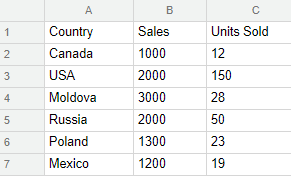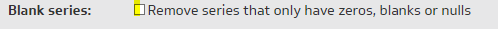Hi Klipfolio,

This post will show you how to alternate between what a series shows you on a chart.
The first method will detail how to do this using just one series, and the second two completely different series.
In both examples, we assume we have a user input control, controlling the variable \$type.

Method 1:
You can use this method when the only difference between the two series is something simple, like the column being selected.
In this case, we have the following datasource:Our X-Axis is the first column, and our series will display column B if the \$type variable is "Sales", and column C if it is not.
The series formula would thus be done using the IF function.
IF(
\$type="Sales",
@B:B,
@C:C
)

Method 2:
This method is for when you have very different formulas for the series, or ones that look at completely different data.
Eg, in this case, for the same datasource as above, we want our series to show either the number of units sold, or the average price per unit.
What we are actually going to do is use two series, and only display one of them.
We again use the IF function to filter our series, however this time we show nothing if the variable is not for the correct series.

-First, our first series is just column @C:C, or nothing
IF(
\$type="Units Sold",
@C:C,
BLANK()
)

-Our second series is column @B:B/@C:C
IF(
\$type="Cost per Unit",
@B:B/@C:C,
BLANK()
)

Then, in the Properties panel of the chart itself, check this box here.Hope this helps you out!

Best,
Joshua